# cffilter

Christiano-Fitzgerald filter for trend and cyclical components

Since R2023a

## Syntax

``[Trend,Cyclical] = cffilter(Y)``
``[TTbl,CTbl] = cffilter(Tbl)``
``[___] = cffilter(___,Name=Value)``
``cffilter(___)``
``cffilter(ax,___)``
``````[___,h] = cffilter(___)``````

## Description

Separate one or more time series into additive trend and cyclical components by applying the Christiano-Fitzgerald filter . `cffilter` optionally plots the series and trend component, with cycles removed.

In addition to the Christiano-Fitzgerald filter, Econometrics Toolbox™ supports the Baxter-King (`bkfilter`), Hamilton (`hfilter`), and Hodrick-Prescott (`hpfilter`) filters.

example

````[Trend,Cyclical] = cffilter(Y)` returns the additive trend `Trend` and cyclical `Cycilcal` components from applying the Christiano-Fitzgerald filter to each variable (column) of the input matrix of time series data `Y`, using the definition of a business cycle in  for quarterly data.```

example

````[TTbl,CTbl] = cffilter(Tbl)` returns the tables or timetables `TTbl` and `CTbl` containing variables for the trend and cyclical components, respectively, from applying the Christiano-Fitzgerald filter to each variable in the input table or timetable `Tbl`. To select different variables in `Tbl` to filter, use the `DataVariables` name-value argument.```

example

````[___] = cffilter(___,Name=Value)` specifies options using one or more name-value arguments in addition to any of the input argument combinations in previous syntaxes. `cffilter` returns the output argument combination for the corresponding input arguments. For example, ```cffilter(Tbl,Symmetric=true,Drift=[false false true],DataVariables=1:3)``` applies the symmetric Christiano-Fitzgerald filter to the first three variables in the input table `Tbl`, and removes the linear drift term from the third variable before applying the filter.```

example

````cffilter(___)` plots time series variables in the input data and their respective smoothed trend components (cycles removed), computed by the Christiano-Fitzgerald filter, on the same axes.```
````cffilter(ax,___)` plots on the axes specified by `ax` instead of the current axes (`gca`). `ax` can precede any of the input argument combinations in the previous syntaxes.```

example

``````[___,h] = cffilter(___)``` plots the specified series and their trend components, and additionally returns handles to plotted graphics objects. Use elements of `h` to modify properties of the plot after you create it.```

## Examples

collapse all

Plot the cyclical component of the US post-WWII, seasonally adjusted, quarterly, real gross national product (`GNPR`).

```load Data_GNP GNPR = Data(:,2); [trend,cyclical] = cffilter(GNPR); T = numel(trend)```
```T = 235 ```

`trend` and `cyclical` are 235-by-1 vectors containing the trend and cyclical components, respectively, resulting from applying the asymmetric Christiano-Fitzgerald filter to the series with default upper and lower cutoffs.

```plot(dates,cyclical) axis tight ylabel("Real GNP Cyclical Component")```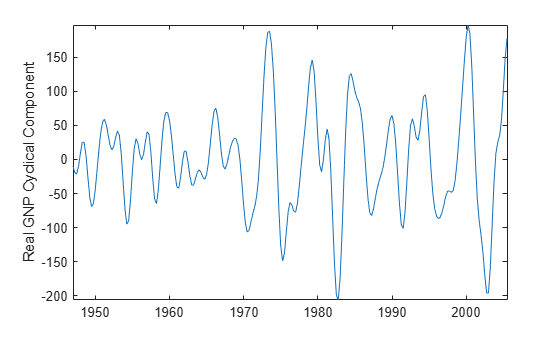Apply the Christiano-Fitzgerald filter to all variables in input table variables.

Load the Schwert stock data set `Data_SchwertStock.mat`, which contains monthly returns of the NYSE index from 1871 through 2008 in `DataTimeTableMth`, among three other variables (for details, enter `Description`). Remove all missing observations from all series.

```load Data_SchwertStock TTM = rmmissing(DataTimeTableMth);```

Aggregate the monthly data in the timetable to quarterly measurements.

`TTQ = convert2quarterly(TTM);`

Apply the asymmetric Christiano-Fitzgerald filter to all variables in the quarterly timetable. Use the default cutoffs.

```[TQTT,CQTT] = cffilter(TTQ); size(TQTT)```
```ans = 1×2 220 4 ```

`TQTT` and `CQTT` are 220-by-4 timetables containing the trend and cyclical components, respectively, of the series in `TTQ`. Variables in the input and output timetables correspond. By default, `cffilter` filters all variables in the input table or timetable. To select a subset of variables, set the `DataVariables` option.

To compare outputs between different tabular inputs, apply the Christiano-Fitzgerald filter to all variables in the table of monthly data `DataTableMth` and the timetable of monthly data `TTM`.

```% Table input of daily data DTM = rmmissing(DataTableMth); [TMDT,CMDT] = cffilter(DTM); size(TMDT)```
```ans = 1×2 656 4 ```
`tail(TMDT)`
``` Return DivYld CapGain CapGainA __________ _________ __________ __________ May1925 0.082825 0.0030391 0.079846 0.079786 Jun1925 0.005878 0.0057145 0.00023547 0.00016356 Jul1925 0.014371 0.0054548 0.0089194 0.0089158 Aug1925 0.046424 0.0033349 0.043012 0.04309 Sep1925 0.022868 0.0064024 0.016375 0.016465 Oct1925 0.079477 0.0058413 0.073612 0.073636 Nov1925 0.00055416 0.003497 -0.0028871 -0.0029429 Dec1925 0.050338 0.0068429 0.043564 0.043495 ```
`tail(CMDT)`
``` Return DivYld CapGain CapGainA __________ ___________ __________ ___________ May1925 -0.016639 -0.00055599 -0.016143 -0.016083 Jun1925 -0.0026377 -0.00025619 -0.0024535 -0.0023816 Jul1925 0.0044847 -0.00024249 0.0047236 0.0047272 Aug1925 0.0017835 -0.00062296 0.0024837 0.0024064 Sep1925 -0.0060897 -0.0011003 -0.0048985 -0.0049894 Oct1925 -0.011281 -0.0012017 -0.010055 -0.010079 Nov1925 -0.0090187 -0.00068252 -0.0083919 -0.0083361 Dec1925 -0.000747 0.00024995 -0.0010665 -0.00099695 ```
```% Timetable input of daily data [TMTT,CMTT] = cffilter(TTM); size(TMTT)```
```ans = 1×2 656 4 ```
`tail(TMTT)`
``` Time Return DivYld CapGain CapGainA ___________ __________ _________ __________ __________ 01-May-1925 0.082825 0.0030391 0.079846 0.079786 01-Jun-1925 0.005878 0.0057145 0.00023547 0.00016356 01-Jul-1925 0.014371 0.0054548 0.0089194 0.0089158 01-Aug-1925 0.046424 0.0033349 0.043012 0.04309 01-Sep-1925 0.022868 0.0064024 0.016375 0.016465 01-Oct-1925 0.079477 0.0058413 0.073612 0.073636 01-Nov-1925 0.00055416 0.003497 -0.0028871 -0.0029429 01-Dec-1925 0.050338 0.0068429 0.043564 0.043495 ```
`tail(CMTT)`
``` Time Return DivYld CapGain CapGainA ___________ __________ ___________ __________ ___________ 01-May-1925 -0.016639 -0.00055599 -0.016143 -0.016083 01-Jun-1925 -0.0026377 -0.00025619 -0.0024535 -0.0023816 01-Jul-1925 0.0044847 -0.00024249 0.0047236 0.0047272 01-Aug-1925 0.0017835 -0.00062296 0.0024837 0.0024064 01-Sep-1925 -0.0060897 -0.0011003 -0.0048985 -0.0049894 01-Oct-1925 -0.011281 -0.0012017 -0.010055 -0.010079 01-Nov-1925 -0.0090187 -0.00068252 -0.0083919 -0.0083361 01-Dec-1925 -0.000747 0.00024995 -0.0010665 -0.00099695 ```

Because the data is disaggregated, the outputs of the daily data have more rows than from the quarterly data. The filter results of the daily inputs are equal among the corresponding outputs, but `cffilter` returns tables of results, instead of timetables, when you supply data in a table.

Load the Nelson-Plosser macroeconomic data set `Data_NelsonPlosser.mat`, which contains series measured yearly in the timetable `DataTimeTable`.

`load Data_NelsonPlosser`

Apply the asymmetric Christiano-Fitzgerald filter to the real and nominal GNP series, `GNPR` and `GNPN`, respectively. Filter out cyclical component frequencies outside the interval [2,8]. Plot the trend component with each series.

```figure cffilter(DataTimeTable,DataVariables=["GNPR" "GNPN"], ... LowerCutoff=2,UpperCutoff=8);```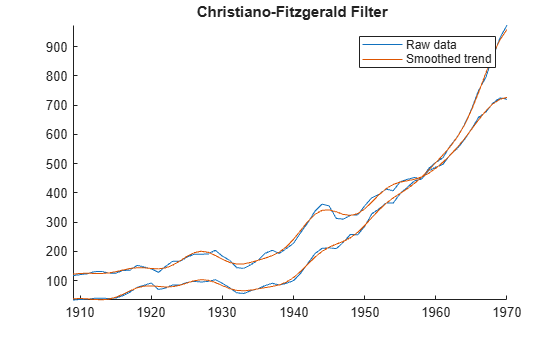Compare the results of the asymmetric filter with the symmetric filter. in addition to cyclical component cutoffs of 2 and 8, and set the lag length of the symmetric moving average to 3 years.

```figure cffilter(DataTimeTable,DataVariables=["GNPR" "GNPN"], ... LowerCutoff=2,UpperCutoff=8,FilterType="symmetric",LagLength=3);```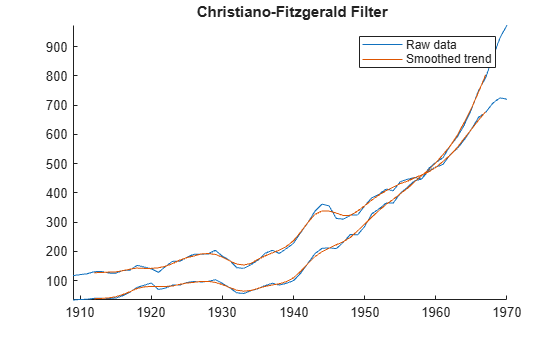Unlike the asymmetric filter, the first and last `LagLength=3` values of the returned components of the the symmetric filter are `NaN`-valued.

Experiment with filter parameter values by adjusting the interactive controls. Because this setup always sets the lag length of the symmetric moving average, `cffilter` implements the symmetric method only.

```varnames = string(DataTimeTable.Properties.VariableNames); lc =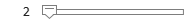2; % LowerCutoff uc =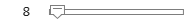8; % UpperCutoff q =3; % LagLength tfs =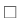false; % Stationarity tfd =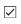true; % Drift vn =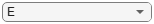varnames(5); % DataVariables figure [TTbl,CTbl,h] = cffilter(DataTimeTable,DataVariables=vn, ... LowerCutoff=lc,UpperCutoff=uc,LagLength=q,Stationarity=tfs, ... Drift=tfd);```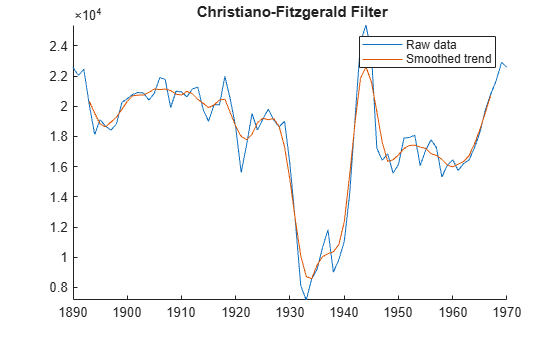## Input Arguments

collapse all

Time series data, specified as a `numObs`-by-`numVars` numeric matrix. Each column of `Y` corresponds to a variable, and each row corresponds to an observation.

Data Types: `double`

Time series data, specified as a table or timetable with `numObs` rows. Each row of `Tbl` is an observation.

Specify `numVars` variables to filter by using the `DataVariables` argument. The selected variables must be numeric.

Axes on which to plot, specified as an `Axes` object.

By default, `cffilter` plots to the current axes (`gca`).

Note

`cffilter` removes, from the specified data, all rows containing at least one missing observation, represented by a `NaN` value.

### Name-Value Arguments

Specify optional pairs of arguments as `Name1=Value1,...,NameN=ValueN`, where `Name` is the argument name and `Value` is the corresponding value. Name-value arguments must appear after other arguments, but the order of the pairs does not matter.

Before R2021a, use commas to separate each name and value, and enclose `Name` in quotes.

Example: ```cffilter(Tbl,Symmetric=true,Drift=[false false true],DataVariables=1:3)``` applies the symmetric Christiano-Fitzgerald filter to the first three variables in the input table `Tbl`, and removes the linear drift term from the third variable before applying the filter.

Lower cutoff period for the cyclical component, in units of data periodicity, specified as a numeric scalar or a length `numVars` vector with elements greater than or equal to `2`.

For a scalar, `cffilter` uses `LowerCutoff` for all input series.

For a vector, `cffilter` applies `LowerCutoff(j)` to selected `j` in the input data.

The default is `6`, meaning 6 quarters (18 months), which is based on the definition of business cycle in .

For more details, see Tips

Example: `LowerCutoff=[4 6]` applies a lower cutoff of `4` to the first series in the input data and a lower cutoff of `6` to the second series.

Data Types: `double`

Upper cutoff period for the cyclical component, in units of data periodicity, specified as a numeric scalar or a length `numVars` vector with elements greater than or equal to the corresponding `LowerCutoff` for each series.

For a scalar, `cffilter` uses `UpperCutoff` for all input series.

For a vector, `cffilter` applies `UpperCutoff(j)` to series `j` in the input data.

The default is `32`, meaning 32 quarters (8 years), which is based on the definition of business cycle in .

For more details, see Tips

Example: `UpperCutoff=[32 36]` applies an upper cutoff of `32` to the first series in the input data and an upper cutoff of `36` to the second series.

Data Types: `double`

Finite-sample approximation of ideal bandpass filter, specified as a value in this table.

ValueDescription
`"asymmetric"`Asymmetric, time-varying moving average
`"symmetric"`Symmetric, time-invariant moving average

If you set `LagLength`, `cffilter` sets `FilterType` to `"symmetric"`.

Example: `FilterType="symmetric"`

Data Types: `char` | `string`

Number of consecutive lags of the moving average for the symmetric filter (see `FilterType`), specified as a positive integer less than `(numObs−1)/2` or vector of such positive integers.

For a scalar, `cffilter` applies `LagLength` to all input series.

For a vector, `cffilter` applies `LagLength(j)` to series `j` in the input data.

If you specify `LagLength`, `cffilter` sets `FilterType` to `"symmetric"`.

The default is `12`, suggested in  for quarterly data.

For more details, see Tips

Example: `LagLength=[4 8]` sets `FilterType` to `"symmetric"` and specifies `4` lags for the symmetric moving average of the first series in the input data and `8` for the symmetric moving average of the second series.

Data Types: `double`

Flag indicating whether input series is stationary, specified as a value or a vector of values in this table.

ValueDescription
`false`Input series is a nonstationary random walk.
`true`Input series is stationary; `cffilter` adjusts the filter to use ideal weights at series endpoints.

For more details on the alternatives, see .

For a scalar, `cffilter` applies `Stationarity` to all input series.

For a vector, `cffilter` applies `Stationarity(j)` to series `j` in the input data.

Example: `Stationarity=[true false]` specifies that the first input series is stationary and the second input series is nonstationary.

Data Types: `logical`

Flag indicating drift in the input series, specified as a value in this table.

ValueDescription
`false``cffilter` does not linear drift from the input series.
`true``cffilter` removes linear drift from the input series before it applies the filter.

For a scalar, `cffilter` applies `Drift` to all input series.

For a vector, `cffilter` applies `Drift(j)` to series `j` in the input data.

Example: `Drift=[false true]` removes the linear drift from only the second input series.

Data Types: `logical`

Variables in `Tbl` that `cffilter` filters, specified as a string vector or cell vector of character vectors containing variable names in `Tbl.Properties.VariableNames`, or an integer or logical vector representing the indices of names. The selected variables must be numeric.

Example: `DataVariables=["GDP" "CPI"]`

Example: `DataVariables=[true true false false]` or `DataVariables=[1 2]` selects the first and second table variables.

Data Types: `double` | `logical` | `char` | `cell` | `string`

## Output Arguments

collapse all

Smoothed trend component τt of each series in the data, returned as a `numObs`-by-`numVars` numeric matrix. `cffilter` returns `Trend` when you supply the input `Y`.

When `cffilter` implements the symmetric filter (see `FilterType`) for a series, the first and last `LagLength` values are `NaN` for the corresponding column in `Trend`.

Cyclical component ct of each series in the data, returned as a `numObs`-by-`numVars` numeric matrix. `cffilter` returns `Cyclical` when you supply the input `Y`.

When `cffilter` implements the symmetric filter (see `FilterType`) for a series, the first and last `LagLength` values are `NaN` for the corresponding column in `Cyclical`.

Smoothed trend component τt of each specified series, returned as a `numObs`-by-`numVars` table or timetable, the same data type as `Tbl`. `cffilter` returns `TTbl` when you supply the input `Tbl`.

For each selected series and corresponding `LagLength`, when `cffilter` implements the symmetric filter (see `FilterType`) for a series, the first and last `LagLength` values are `NaN` in the corresponding variable in `TTbl`.

Cyclical component ct of each specified series, returned as a `numObs`-by-`numVars` table or timetable, the same data type as `Tbl`. `cffilter` returns `CTbl` when you supply the input `Tbl`.

For each selected series and corresponding `LagLength`, when `cffilter` implements the symmetric filter (see `FilterType`) for a series, the first and last `LagLength` values are `NaN` in the corresponding variable in `CTbl`.

Handles to plotted graphics objects, returned as a vector of graphics objects. `cffilter` plots the data and trend only when you return no outputs or you return `h`.

`h` contains unique plot identifiers, which you can use to query or modify properties of the plot.

collapse all

### Christiano-Fitzgerald Filter

The Christiano-Fitzgerald filter separates a time series yt into a trend component τt (`Trend` and `TTbl`) and cyclical component ct (`Cyclical` and `CTbl`) such that yt = τt + ct.

Depending on the value of the value of the `FilterType` name-value argument, `cffilter` implements either an asymmetric, time-varying, or a symmetric, time-invariant moving average as a finite-sample approximation of an ideal bandpass filter .

## Tips

The definition of a business cycle in  suggests values in the table for the cutoff periods `LowerCutoff` and `UpperCutoff`, and lag length `LagLength` that depend on the periodicity of the data.

Periodicity`LowerCutoff``UpperCutoff``LagLength`
Yearly283
Quarterly63212
Monthly189636

In practice, use vectors of cutoff periods and lag lengths to test alternatives. Use the plot produced by `cffilter` to compare results among settings.

 Burns, Arthur F., and Wesley C. Mitchell. Measuring Business Cycles. Cambridge, MA: National Bureau of Economic Research, 1946.

 Christiano, Lawrence J., and Terry J. Fitzgerald. "The Band Pass Filter." International Economic Review 44 (May 2003): 435–65. https://doi.org/10.1111/1468-2354.t01-1-00076.